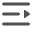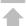# C++ BSON 使用

## C++ BSON 主要类

• bson::BSONObj：创建 BSONObj 对象。

• bson::BSONElement：BSONObj对象由 BSONElement 对象组成，即 BSONElement 对象为 BSONObj 对象的字段或者元素，它是键值对。

• bson::BSONObjBuilder：BSONObjBuilder 用来实例化 BSONObj 对象。

• bson::BSONObjlterator：BSONObjlterator 用来遍历 BSONObj 对象中的元素。

• typedef bson::BSONElement be;

• typedef bson::BSONObj bo;

• typedef bson::BSONObjBuilder bob;

## 建立对象

• 使用 BSONObject，BSONObjBuilder 建立对象

```#include "client.hpp"

using namespace bson ;
BSONObj obj ;
BSONObjBuilder b ;

b.append("name","sam") ;
b.append("age","24") ;
obj = b.obj() ;
或者
obj = BSONObjBuilder().genOID().append("name","sam").append("age",24).obj() ;```

另外，可以使用数据流的方法建立 BSONObj 对象。

```BSONObj obj ;
BSONObjBuilder b ;
b << "name" << "sam" << "age" << "24" ;
obj = b.obj() ;```
• 使用宏 BSON 建立对象

C++ BSON 中定义还定义了一个 BSON 的宏，可以用它来快速地建立 BSONObj 对象。

```BSONObj obj ;
// int
obj = BSON( "a" << 1 ) ;
// float
obj = BSON( "b" << 3.14159265359 ) ;
// string
obj = BSON( "sample" << "employee" ) ;
// OID
obj = BSON( GENOID ) ;
// bool
obj = BSON( "flag" << true << "ret" << false ) ;
// object
obj = BSON( "d" << BSON("e" << "hi!") ) ;
// array
obj = BSON( "phone" << BSON_ARRAY( "13800138123" << "13800138124" ) ) ;
// others, less then, greater then, etc
obj = BSON( "g" << LT << 99 ) ;```
• 使用 fromjson 接口建立对象

此外，可以使用 fromjson.hpp 中的 fromjson() 将 json 字符串转换成 BSONObj 对象。

```string s("{name:\"sam\"}") ;
fromjson ( s, obj ) ;
或者
const char *r ="{ \
firstName:\"Sam\", \
lastName:\"Smith\",age:25,id:\"count\", \收起